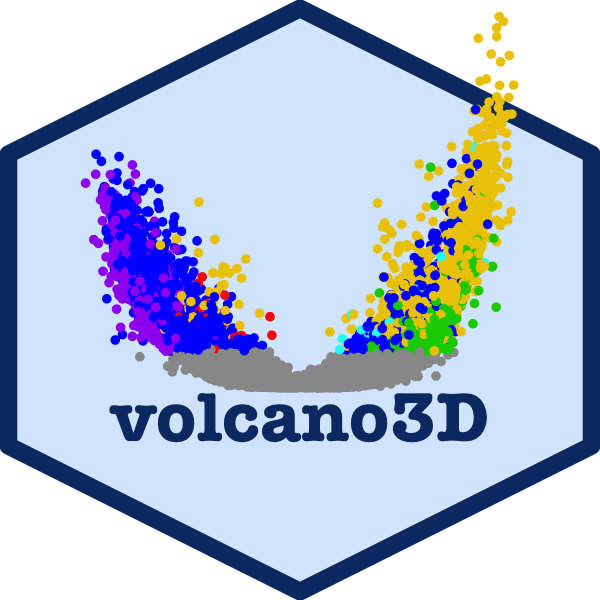## 2x3-way analysisThe main work flow for using volcano3D is ideal for comparing high dimensional data such as gene expression or other biological data across 3 classes, and this is covered in the main vignette. However, an alternative use of 3-way radial plots and 3d volcano plots is for 2x3-way analysis. In this type of analysis there is a binary factor such as drug response (responders vs non-responders) and a 2nd factor with 3 classes such as a trial with 3 drugs.

## Example

This vignette shows analysis from the STRAP trial in rheumatoid arthritis, in which patients were randomised to one of three drugs (etanercept, rituximab, tocilizumab). Clinical response to treatment (a binary outcome) was measured after 16 weeks. Gene expression data from RNA-Sequencing (RNA-Seq) of synovial biopsies from the patients’ inflamed joints was performed at baseline. RNA-Seq data is count based and overdispersed, and thus is typically best modelled by a negative binomial distribution.

## DESeq2 pipeline

Differential gene expression to compare the synovial gene expression between responders vs non-responders is performed using the Bioconductor package DESeq2. Since there are 3 distinct drugs this becomes a 2x3-factor analysis.

The following code shows set up and 2x3-way analysis in DESeq2 followed by generation of a ‘volc3d’ class results object for plotting and visualisation of the results.

``````library(volcano3D)

# Basic DESeq2 set up
library(DESeq2)

counts <- matrix(rnbinom(n=3000, mu=100, size=1/0.5), ncol=30)
rownames(counts) <- paste0("gene", 1:100)
cond <- rep(factor(rep(1:3, each=5), labels = c('A', 'B', 'C')), 2)
resp <- factor(rep(1:2, each=15), labels = c('non.responder', 'responder'))
metadata <- data.frame(drug = cond, response = resp)

# Full dataset object construction

# Perform 3x DESeq2 analyses comparing binary response for each drug
res <- deseq_2x3(dds, ~response, "drug")``````

The design formula can contain covariates, however the main contrast should be the last term of the formula as is standard in DESeq2 analysis.

The function `deseq_2x3()` returns a list of 3 DESeq2 objects containing the response analysis for each of the three drugs. These response vs non-response differential expression comparisons can be quickly visualised with the `easyVolcano()` function from the R package `easylabel`, which is designed for DESeq2 and limma objects and uses an interactive R/shiny interface.

``````library(easylabel)
df <- as.data.frame(res[])  # results for the first drug
easyVolcano(df)``````

The `deseq_2x3` output is passed to `deseq_2x3_polar()` to generate a `volc3d` class object for plotting. Thus the 3-way radial plot and 3d volcano plot simplify visualisation of the 2x3-way analysis, replacing 3 volcano plots with a single radial plot or 3d volcano plot.

``````# Generate polar object
obj <- deseq_2x3_polar(res)

# 2d plot
The example below using real data from the STRAP trial demonstrates a 3-way radial plot highlighting the relationship between genes significantly increased in responders across each of the three drugs. The pipe to `toWebGL()` is used to speed up plotting by using webGL instead of SVG in plotly scatter plots. Alternatively, a ggplot2 version can be plotted using `radial_ggplot()`.
``````obj <- deseq_2x3_polar(data1)